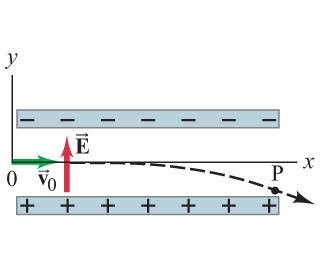# Electrion moving between two parallel plates

• toothpaste666
In summary, the angle at which the electrons will leave the electric field at the end of the parallel plates is -13 degrees.

## Homework Statement

At what angle will the electrons in the image leave the uniform electric field at the end of the parallel plates (point P) ? Assume the plates are .049 m long, E = 5x10^3 N/C and v0 = 1x10^7 m/s. Ignore fringing of the field.E = kQ/r^2
F=ma
F= kQ1Q2/r^2

## The Attempt at a Solution

I am having difficulty figuring out how to get started. I know the equation for the motion of the electron is
y= -(eEx^2)/(2mv0^2) but I don't know if this helps me. I think they want the angle the path of the electron makes with the x axis, but I am not sure. Can someone get me started or give me a hint on how to relate the angle to everything else please?

The angle at which the electron is moving is given by the direction of the velocity vector of the electron. So, try to figure out the x and y components of the velocity at point P.

Note: Two out of the three equations that you listed under "relevant equations" are not actually relevant to this problem. Can you spot them?

I tried plugging in .049 m for x into the equation of motion as well as the mass of an electron for m and all the other given information and got
y = - [(1.602x10^-19)(5x10^3)(.049)^2]/[2(9.1x10^-31)(1x10^7)^2] = -.011 m
which is how far below the x-axis it is so the angle would be tan^-1(-.011/.049) = -13 degrees?

I guess F= ma is the relevant one?

toothpaste666 said:
I guess F= ma is the relevant one?
Right. But you'll need some other equations, too.

ok so to find the velocity vector at point P ... there is no x component of acceleration, so vx= v0
vy = vy0 - at
vy0 = 0 so
vy = - at
a = F/m = qE/m = -eE/m
so
vy = eEt/m ... am i on the right track?

toothpaste666 said:
ok so to find the velocity vector at point P ... there is no x component of acceleration, so vx= v0
vy = vy0 - at
vy0 = 0 so
vy = - at
a = F/m = qE/m = -eE/m
so
vy = eEt/m ... am i on the right track?

Yes, you are!

I think I am now stuck though because I don't have any information for t

Any way to use the information about the length of the plates?

ah thank you! so it constantly travels 1x10^7 m/s in the x direction for the length of .049 m.
v = d/t so t= d/v = .049 m / 1x10^7 m/s = 4.9 x 10 ^-9 s
so
vy = eEt/m = (1.602x10^-19)(5x10^3)(4.9x10^-9)/(9.1x10^-31) = 4313077 m/s right?

That looks right. I haven't actually grabbed a calculator and checked your numbers.

ok so that means theta = tan^-1(4313077/(1x10^7)) = 23.3 degrees but -23.3 degrees because it is going clockwise from the x axis

Yes. Or you can just say 23.3o below the horizontal. (That way, whoever sees your answer doesn't have to decipher the meaning of the negative sign.) But, if you are having to plug your answer into some sort of grading program, then you might need to include the sign.

•toothpaste666
thank you so much!